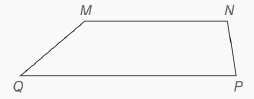Chapter P.CR, Problem 36CRElementary Geometry For College St...

7th Edition
Alexander + 2 others
ISBN: 9781337614085

Solutions

Chapter
SectionElementary Geometry For College St...

7th Edition
Alexander + 2 others
ISBN: 9781337614085
Textbook Problem

Given that MNPQ is a “trapezoid”, use intuition to draw a conclusion regarding M N - and P Q -To determine

To find:

A conclusion regarding measures of MN- and PQ- using intuition.

Explanation

Approach:

Reasoning:

Reasoning is a process on experience and principles that leads to a conclusion.

Intuition:

We often say “It occurs to me that...” With intuition, a sudden insight allows one to make a statement without applying any formal reasoning. When intuition is used, we sometimes make an error by jumping to conclusions.

Parallel lines:

Parallel lines lie on the same page and will not cross over each other even if they are extended infinitely.

Procedure:

It is given that MNPQ is a “trapezoid”.

By observing the given diagram, it can be seen that MN- and PQ- will not cross over each other even if they are extended infinitely

Still sussing out bartleby?

Check out a sample textbook solution.

See a sample solution

The Solution to Your Study Problems

Bartleby provides explanations to thousands of textbook problems written by our experts, many with advanced degrees!

Get Started

15. Explain the difference between an ordinary annuity and an annuity due.

Mathematical Applications for the Management, Life, and Social Sciences

Describe the scores in a sample that has standard deviation of zero.

Statistics for The Behavioral Sciences (MindTap Course List)

Simplify: 3064

Elementary Technical Mathematics

Express the limit as a derivative and evaluate. 108. lim/3cos0.5/3

Single Variable Calculus: Early Transcendentals, Volume I

In Exercises 110, find the graphical solution to each inequality. xy0

Finite Mathematics for the Managerial, Life, and Social Sciences

True or False: If a function is not increasing on an interval, then it is decreasing on the interval.

Study Guide for Stewart's Single Variable Calculus: Early Transcendentals, 8th